Courses

# Test: Design Of Joints (Level - 3)

## 20 Questions MCQ Test Machine Design | Test: Design Of Joints (Level - 3)

Description
This mock test of Test: Design Of Joints (Level - 3) for Mechanical Engineering helps you for every Mechanical Engineering entrance exam. This contains 20 Multiple Choice Questions for Mechanical Engineering Test: Design Of Joints (Level - 3) (mcq) to study with solutions a complete question bank. The solved questions answers in this Test: Design Of Joints (Level - 3) quiz give you a good mix of easy questions and tough questions. Mechanical Engineering students definitely take this Test: Design Of Joints (Level - 3) exercise for a better result in the exam. You can find other Test: Design Of Joints (Level - 3) extra questions, long questions & short questions for Mechanical Engineering on EduRev as well by searching above.
QUESTION: 1

### Assertion (A): Uniform-strength bolts are used for resisting impact loads.Reason (R): The area of cross-section of the threaded and unthreaded parts is made equal

Solution:

Uniform-strength bolts are used for resisting impact loads as these bolts have more strain energy storing capacity.

⇒The area of cross-section of the threaded and unthreaded portion is made equal to increase the strain energy storing capacity.

QUESTION: 2

### Two plates are joined together using two bolts as shown in figure. The shear strength in yield for the bolt material is N mm . Taking a factor of safety of 6, the diameter of the bolt is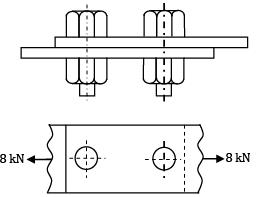Solution: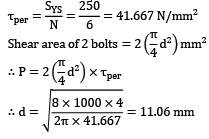QUESTION: 3

### A circular shaft, 40 mm in diameter, is welded to the support by means of circumferential fillet weld. It is subjected to a torsional moment of 3000 Nm. Permissible shear stress in weld is limited to N mm . The size of weld is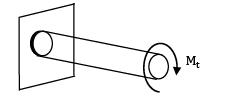Solution:

Torsional shear stress in the weld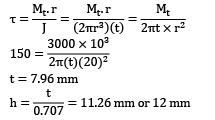t = 7.96 mm*Answer can only contain numeric values
QUESTION: 4

A plate 50 mm wide and 10 mm thick is to be welded as T joint to another plate by means of two parallel fillet welds. If the plates are subjected to a static load of 78 kN and the allowable shear stress is 144 MPa. The weld length per side (in mm

Solution: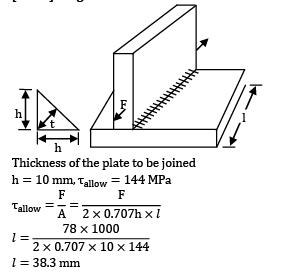Thickness of the plate to be joined

h = 10 mm,τallow = 144 MPal = 38.3 mm

*Answer can only contain numeric values
QUESTION: 5

A steel plate 200 mm wide and 5 mm thick is welded with another steel plate as shown in figure. The permissible tensile and shear stresses for the weld material are 80 MPa and 60 MPa respectively. If the plates can withstand a load upto 80 kN, then the ratio of the strength of transverse fillet weld to the double parallel fillet weld is __________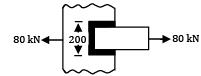Solution:

Let, P1 = Strength of transverse fillet weld

P2 = Strength of double parallel fillet weld

Now, P1 = 0.707 (hl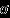t)

= 0.707 x 5 x 200 x 60

= 42420 N

Now, P = P1 + P2

P2 = P - P1 = 80000 - 42420 = 37580 N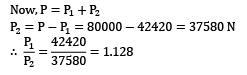QUESTION: 6

A bracket shown in the figure is bolted to a column using two bolts. If the permissible shear stress of the bolt material is 300 MPa, then the factor of safety is ( a ing d mm)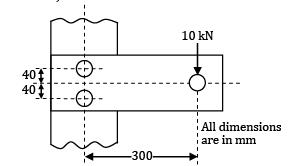Solution: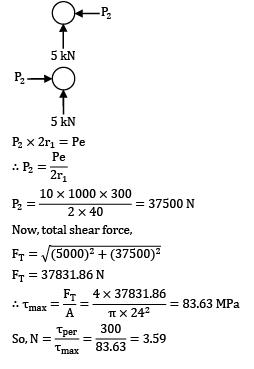P2 x 2r1 = PeNow, total shear force,FT = 37831.86 NQUESTION: 7

A riveted joint has been designed to support an eccentric load P. The load generates value of equal to 4 kN and equal to 3 kN. The cross-sectional area of each rivet is mm . Consider the following statements

1. The stress in the rivet is N mm

2. The value of eccentricity L is 100 mm

3. The value of load P is 6 kN

4. The resultant force in each rivet is 6 kN Which of these statements are correct?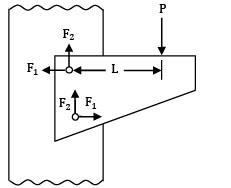Solution: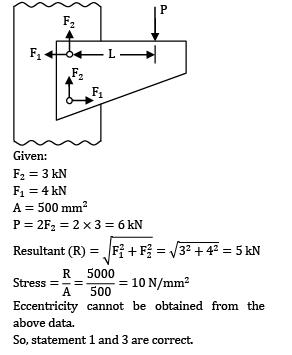Given:

F2 = 3 kN

F1 = 4 kN

A = 500 mm2

P = 2F2 = 2 x 3 = 6 kNEccentricity cannot be obtained from the above data.

So, statement 1 and 3 are correct.

QUESTION: 8

For the figure shown below, the permissible tensile and shear stresses for the weld material and plates are N mm and N mm . If the depth of weld is 15 mm, the length of each parallel fillet weld is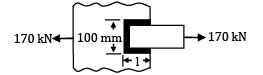Solution:

Strength of transverse fillet weld P1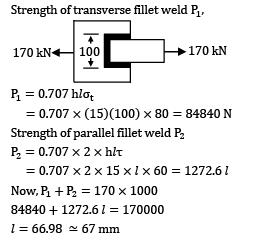P1 = 0.707 hlt

= 0.707 x (15)(100) x 80 = 84840 N

Strength of parallel fillet weld P2

P2 = 0.707 x 2 x hlτ

= 0.707 x 2 x 15 x l x 60 = 1272.6 l

Now, P1 + P2 = 170 x 1000

84840 + 1272.6 l = 170000

l = 66.98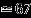mm

QUESTION: 9

A bracket is welded to the vertical plate by means of two fillet welds as shown in figure. The stresses induced in the weld are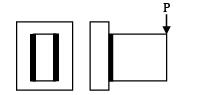Solution:

The load is transverse shear load and it will induce shear force and a bending moment.

QUESTION: 10

Two plates, 25 mm thick, are welded together by means of a reinforced butt weld and subjected to a completely reversed axial load of 土100kN as shown in figure. The throat of the weld is 25 mm. The ultimate tensile strength of the weld metal is 450N/mm2. The surface finish of the weld is equivalent to that of forged component and the reliability is 90%. The length of the weld is

[Assume factor of safety is 2.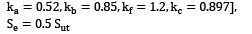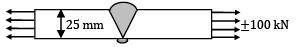Solution:

Given, P =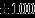kN, h = 25 mm

Sut = 450N/mm2, R = 90%, FOS = 2

Endurance limit, Se = ka,kb,kc,kd,S’e

For 90% Reliability,kc = 0.897

kd = Modifying factor to account for stress concentration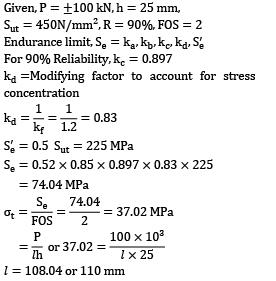S’e = 0.5 Sut = 225 MPa

Se = 0.52 x 0.85 x 0.897 x 0.83 x 225

= 74.04 MPal =108.04 or 110 mm

QUESTION: 11

A circular beam, 50 mm in diameter, is welded to a support by means of a fillet weld as shown in figure. The size of the weld, if the permissible shear stress in the weld is limited to 100N/mm2, is (in mm)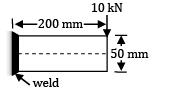Solution:

Given, P = 10 kN,T = 100 N/mm2

Primarily shear stress (T1)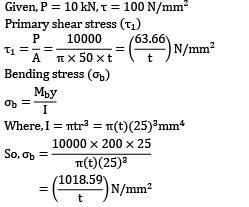Bending stress (b)Where, I = πtr3 = π(t)(25)3mm4Now, maximum shear stress in the weld is;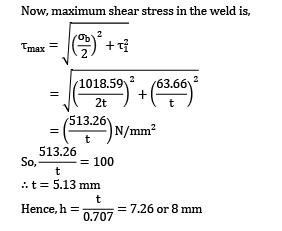∴ t = 5.13 mmQUESTION: 12

A bracket, as shown in figure, is welded to a plate. The welds are of same size

The value of permissible force per mm of the weld length is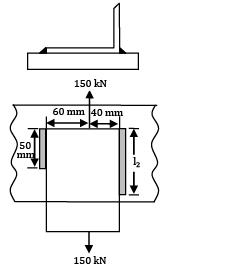Solution: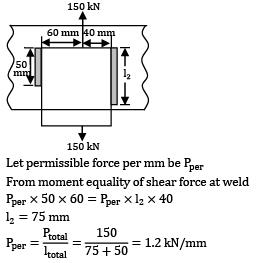Let permissible force per mm be Pper

From moment equality of shear force at weld

Pper x 50 x 60 = Pper x l2 x 40

l2 = 75 mm*Answer can only contain numeric values
QUESTION: 13

A bracket is welded to the vertical plate by means of two fillet welds as shown in the fig. If the permissible shear stress is limited to 55N/mm2 . The size of weld is __________ (mm)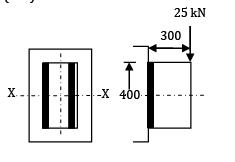Solution:

Given, P = 25 kN

T = 55 N/mm2

Primary shear stress

The total area of two vertical welds is given by,

A = 2(400 t) = (800 t) mm2

The primary shear stress in the weld is given by,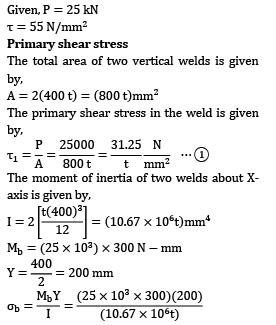The moment of inertia of two welds about Xaxis is given by,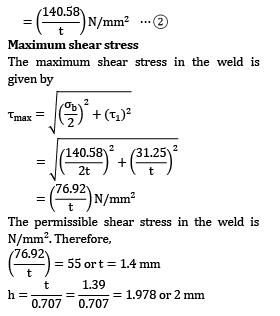Maximum shear stress

The maximum shear stress in the weld is given byThe permissible shear stress in the weld is N/mm2. Therefore,QUESTION: 14

In the figure a plate is connected to another plate by fillet welds around the ends and also inside the machined slot. The working stresses for the transverse welds and longitudinal welds are. 102.5 MN/m2 and 84MN/m2 respectively. If joint is subjected to a pull of 100 kN, the size of weld will be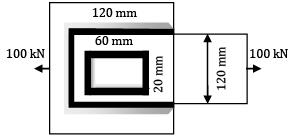Solution:

Length of longitudinal weld,

l = (2 x 120) + (2 x 60) = 360 mm

Length of transverse weld = 120 + 2 x 20

= 160 mm

s = size of weld

∴Effective thickness of weld

t = 0.707 s

Using the relation P =lt

P1 = 360 x 0.707 s x 10-6 x 84 x 106

= 21379 s

Similarly

P2 = 160 x 0.707 s x 10-6 x 102.5 x 106

11595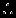Total force of resistance of the welds

P = P1 + P2

= 21379 s + 11595 s

= 32974 s

Now, P = 100 x 103 N

100 x 103 = 32974 s

s = 3.03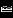3 mm

QUESTION: 15

An mm angle is welded to a steel plate by means of fillet-welds as shown in the figure. The angle is subjected to a static load of 140 kN, which passes through the center of gravity G and the allowable load per mm of the weld length is 700 N. The length of the shorter fillet weld is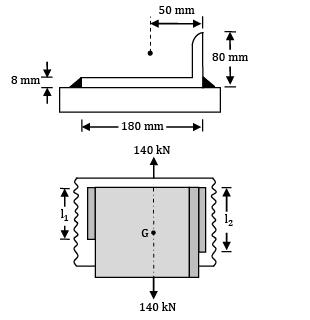Solution:

Total length of weld (l),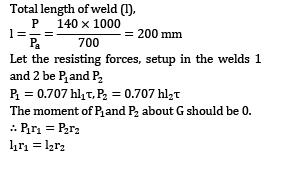Let the resisting forces, setup in the welds 1 and 2 be P1 and P2

P1 = 0.707 hl1T,P2 = 0.707 hl2T

The moment of about G should be 0.

∴ P1r1 = P2r2

l1r1 = l2r2

Also, l1 + l2 = I = 200 mm

l1 x 130 = l2 x 50

L2 = 2.611

L1 = 55.56 mm and l2 = 144.44 mm

QUESTION: 16

The following two figures show welded joints (x x x x x indicates welds), for the same load and same dimensions of plate and weld.

The joint shown in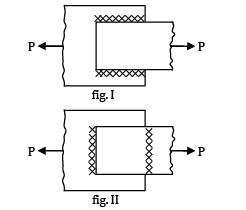Solution:

Figure II is better because the weld is in tension and safe stress of weld in tension is greater than shear.

QUESTION: 17

A plate 1 m long, 70 mm thick is welded to another plate at right angles to each other by 12 mm fillet weld, as shown in the figure. What is the maximum torque T, that the welded joint can sustain if the permissible shear stress intensity in the weld is not exceed to 80 MPa.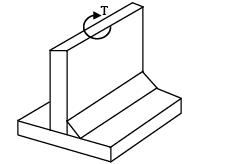Solution: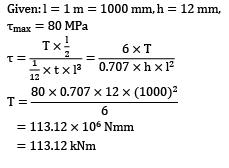QUESTION: 18

A cast iron bracket, supporting the transmission shaft and the belt pulley, is fixed to the steel structure by means of four bolts as shown in Fig. There are two bolts at

A and two bolts at

B. The tensions in slack and tight sides of the belt are 5 kN and 10 kN respectively. The belt tensions act in a vertically downward direction. The distances are as follows,

l1 = 50 mm, l2 = 150 mm, l = 200 mm The maximum permissible tensile stress in any bolt is 60 N/mm2 . Determine the size of the bolts (in mm2 )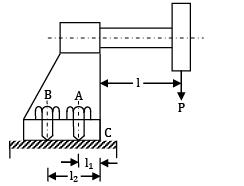Solution:

Given,P = (5 + 10) kN,

l = 200 mmt)max = 60 N/mm2

Tensile stress due to the tendency of bracket to tilt about ‘C’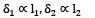P1= Cl1, P2 = Cl2

Pl = 2P1l1 + 2P2l2 = 2(Cl1)l1 + 2(Cl2)l2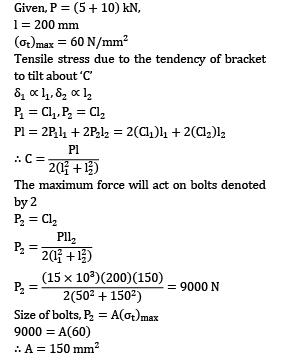The maximum force will act on bolts denoted by 2

P2 = Cl2Size of bolts, P2 = A(t)max

9000 = A(60)

∴A = 150 mm2

QUESTION: 19

Two flat plates subjected to a tensile force p are connected together by means of 25 mm diameter rivets with double strap butt joint as shown.

The thickness of plate is 25 mm and plate width is 200 mm. Rivets and plates are made of same material and having following properties.τper.= 60 MPa, (σt) = 70 MPa, (σc)per= 100 MPa What is the efficiency of the joint?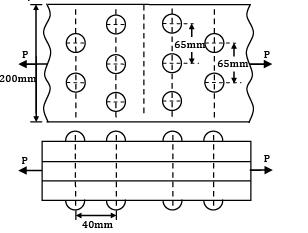Solution:

Given, w = 200 mm,t = 70 MPa,c = 100 MPa

d = 25 mm,T =60 MPa, N =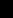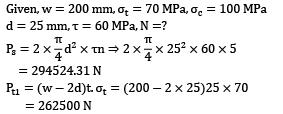= 294524.31 N

Pt1 = (w - 2d)t,t = (200 - 2 x 25)25 x 70

= 262500 N

Pc = dtσcn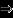25 x 25 x 100 x 5

312500 N

Plate strength, P = wtσt200 x 25 x 70

= 350000 N

Strength of second row,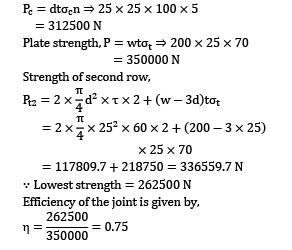X 25 x 70

= 117809.7 + 218750 = 336559.7 N

∵ Lowest strength = 262500 N

Efficiency of the joint is given by,QUESTION: 20

A steel plate is joined with another plate using weld fillet as shown in the figure. If the permissible shear stress of weld material is 50 N/mm2 and leg of the weld is 10 mm, what could be the maximum value of P?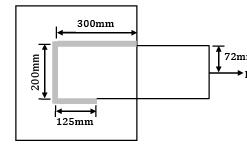Solution:

There will be primary shear stress due to P and secondary shear stress due to moment.

Center of gravity of fillet weld from upper length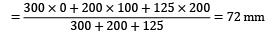As P is applied exactly at center of gravity of fillet weld, there won’t be any moment.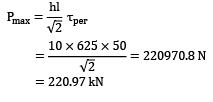= 220.97 kN